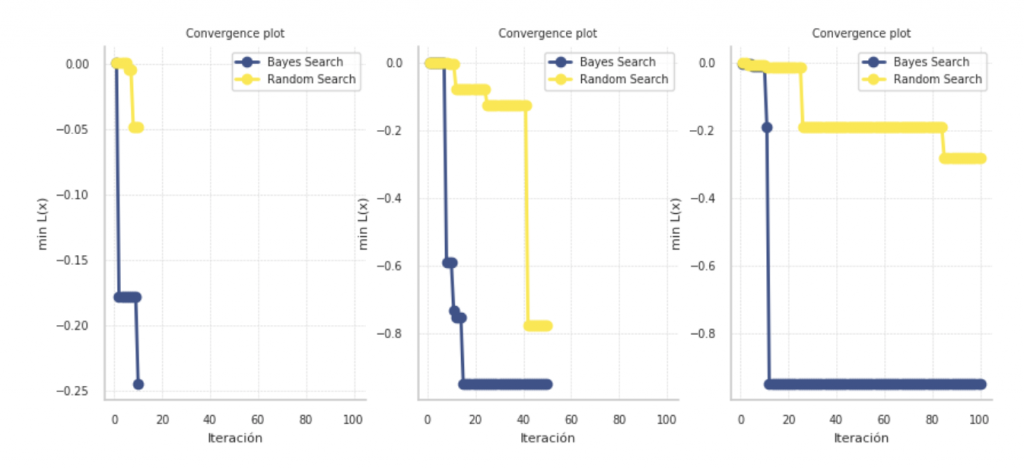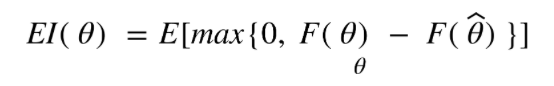Wine is the most consumed alcoholic beverage in the world. This is nothing new; there are references about winegrowing from the Neolithic period.  From then it grew to such a point that in the Greek and Roman cultures there was a god of wine (Bacchus or Dionysius). Today there are numerous regions across the planet where excellent wines are created.

Nevertheless and in spite of the long tradition surrounding wine, decisions must always be made at the moment of production.  Let’s imagine that we are producers who wish to make an exquisite wine.  We will have to choose fermentation time, maturation time, temperature. It would be ideal to try all the possible combinations and see the results, would it not?  However, this option is not possible; we are limited by time (some wines take years to mature) and we probably have budgetary restraints too.

### BUT WHAT DOES THIS HAVE TO DO WITH THE WORLD OF DATA?

Well, what we have just seen is a use case of hyperparameter optimisation.  In Machine Learning we constantly face this issue.  When generating a predictive model (deciding the best way to make wine), we need three things: data (vine, grapes), cost function (time restrictions, money, installations) and hyperparameters (identification of restrictions, selection of limits).

To clarify, parameters must not be confused with hyperparameters.  The difference is that parameters are “learnt” (hence the name ‘machine learning’) through mathematical adjustment that underlies the chosen model with data (the coefficients of a regression, for example) while hyperparameters cannot be learnt in the adjustment process and are properties of the model that the data scientist must choose to reach the objective. It is a process of trial and error, hardly intuitive as problems of many dimensions are often encountered.  Examples of hyperparameters:

• Learning rate in the optimisation of a descending gradient, for example
• Number of trees in a random forest
• Number of layers in a neural network

### FROM MAGIC TO RANDOMNESS…

Let’s formally define the problem:Our optimal hyperparameter vector comes from minimising the generalised error F for our x-sampling from an underlying Gx distribution. This error depends on cost function L, under a training algorithm A optimised for the hyperparameter α and the training data xtrain subspace. By solving this equation, we arrive at:Where ψ is an uknown response function. To solve this equation, we will have to evaluate the possibilities of the Η hyperparameter space until we find the optimal vector of α.

The critical point is therefore to explore this hyperparameter space. What forms are there? Are they all equally efficient?

Intuition tells us that we would have to test each of the possible combinations of hyperparameters. However, this solution, commonly called Grid Search, is computationally very inefficient, due to the problem of dimensionality, that is, the number of possible combinations grows exponentially with the number of hyperparameters. Just as the winemaker does not have enough time to explore all possible avenues of processing, we, as data scientists, lack infinite computational capacity.Grid search graph. Source: Keepler.

On the other hand, there are arguments in favour of this technique:

• We would better understand the response function (we would understand our model’s sensitivities to hyperparameters better).
• Paralleling the process is trivial.
• It is easy to implement.

However, there is also another type of search that is faster and more efficient, called Random Search. The reason why this search algorithm is better than the former is that the response function is more sensitive to changes in some dimensions than others. By exploring the combination space in a random way, we find combinations that would not occur with a uniform search (grid search) because in a uniform search the hyperparameters are equidistant from each other. With a random search, the exploratory space is covered more unevenly, finding areas we would not with a uniform search. If we knew a priori the areas that would be more minimised by the model, we would make an appropriate grid search on those areas, but as we do not generally know this, we must explore all the space.

The following image shows this phenomenon with an experiment. Under a set of randomly generated data (but with statistical meaning), a predictive model is run twice for each dimension of complexity (first experiment: 10, second: 50), and the times and error are compared. The number of computations executed in each search is exactly the same in order to measure the difference in execution time.Experiment on hyperparametric models. Source: Keepler.

As we can see, the time is shorter as we add hyperparameters to space, sometimes up to 50% faster. Although with a dimensionality of 10, the first experiment was not in line with our hypothesis, the rest of the experiments were. It should be noted that the mean of the error is also slightly lower (the distribution is more to the left) in the random search. Therefore, our study will never be distorted if we use a random search, but will gain efficiency!

### …TO THE POWER OF KNOWLEDGE

There are other very powerful techniques that make use of the information generated in each search iteration to know which space area to go to, building a probabilistic model on the cost function. This method is called Bayesian optimisation, and should be applied in situations where our machine learning algorithm does not have closed form, and its solution is reached through an optimisation that is generally expensive to evaluate.Bayesian optimisation. Source: Keepler.

Let’s interpret this graph. Given an Expected Improvement utility function,In each iteration, we:

• Evaluate the cost function with hyperparameters.
• Update our “belief” with a Gaussian process.
• Given our new belief we can use the utility function to determine new hyperparameters in areas where the uncertainty (or standard deviation) of the Gaussian process is lower. That is, we obtain a new better optimal hyperparameter vector.
•